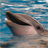# Multiple distance calculation with formula calculation for each

658
1
08-24-2013 05:54 PMby
New Contributor
Ok my task I've metioned here becomes a little more sophisticated. First I've attached a graphic to explain it.

I've got two Layers - shown as blue and red containing like 10.000 polygons each.

I need to calculate for every blue polygon one value. This value is calculated by the distance (Distance 1) between one blue polygon (Area 1) and one red polygon with a unique value (Value 1) --> Value 1 needs to be divided by Distance 1 --> and the result is a factor (X1). This must be done with all other red polygons (10.000 times). --> So finaly I've got one faktor for each Area 1 - red polygon connection - so 10.000 factos. For these factors the average value must be calculated. So far so good - it's just one blue polygon. BUT I've got like 9.999 other where exactly the same needs to be done.

I hope I've explained my problem understandable :rolleyes:

What do you think I should do? Can you help me?
Tags (3)